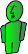# Continuous Release into the Air

The three dimensional nature of our atmosphere often makes tracking airborne pollutants very difficult. This section of Fate allows you to estimate the concentration of a pollutant at a specific distance downwind from a continually releasing source. An example of a source that might release this type of pollutant is a cooling stack on a a pulp mill.

Step 1 Manually convert input data to metric units: meters, grams, and seconds.
Step 2: Enter or calculate the release height

Use the calculated value if the pollutant temperature is higher than the air temperature.

$ΔH_r = \frac{ū_sd}{ū}(1.5 + 2.68 * 10^{-3}Pd\frac{T_s - T_s}{T_s})$
Step 3: Enter the remaining data
Step 4: Choose atmospheric stability
Day Radiation Intensity Night Cloud Cover
Wind Speed (m/s) Strong Medium Slight Cloudy Calm and Clear
< 2 A A-B B
2-3 A-B B C E F
3-5 B B-C C D E
5-6 C C-D D D D
> 6 C D D D D
Step 5: Enter the dispersion coefficients

#### Vertical

A:
B:
C:
D:
E:
F:
A:
B:
C:
D:
E:
F:
Concentration calculations

Result: g/m3

Graph varying distance in Y
$C_{(x,y,z)} = \frac{Q_m}{2\pi\sigma_y\sigma_zu}e^{(-\frac{1}{2}(\frac{y}{\sigma_y})^2)}(e^{(-\frac{1}{2}(\frac{z-H_r}{\sigma_z})^2)}+e^{(-\frac{1}{2}(\frac{z+H_r}{\sigma_z})^2)})$Graph varying distance in Z
$C_{(x,y,z)} = \frac{Q_m}{2\pi\sigma_y\sigma_zu}e^{(-\frac{1}{2}(\frac{y}{\sigma_y})^2)}(e^{(-\frac{1}{2}(\frac{z-H_r}{\sigma_z})^2)}+e^{(-\frac{1}{2}(\frac{z+H_r}{\sigma_z})^2)})$